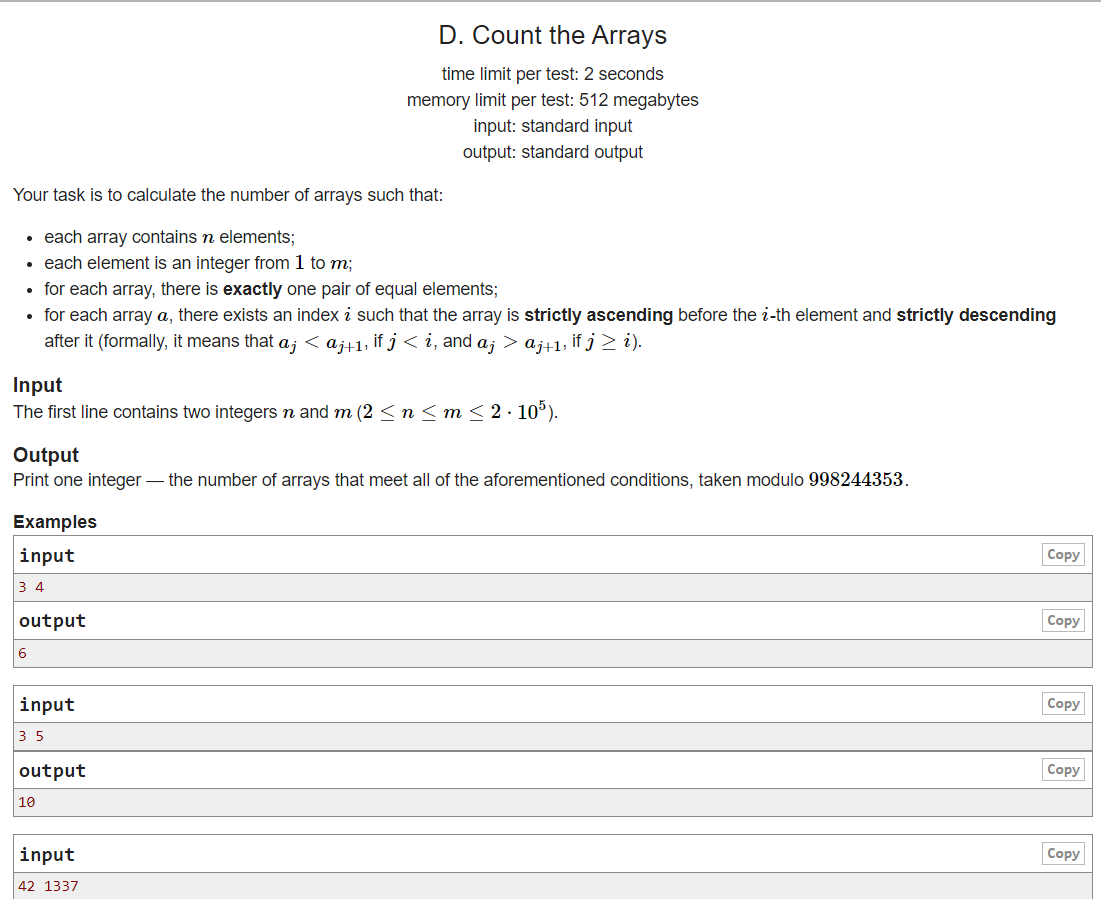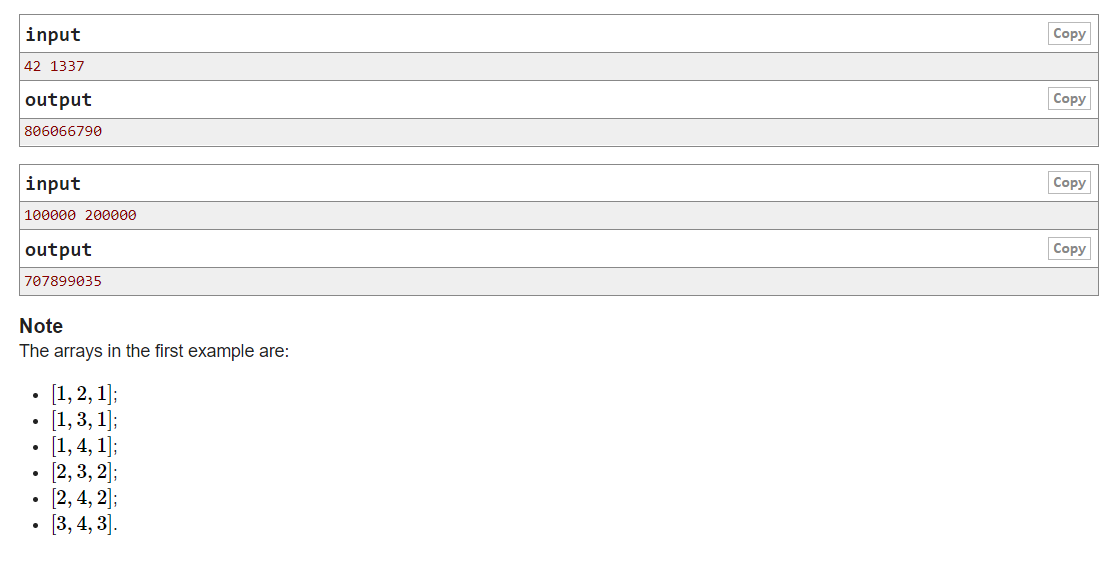## D. Count the Arrays(组合数学+卢卡斯定理)``````#include<bits/stdc++.h>
using namespace std;

typedef long long int ll;
const ll mod = 998244353 ;
ll f;
ll fastpow(ll a,ll b){
ll ans = 1;
while(b>0){
if(b&1)ans = ans * a % mod;
a = a * a % mod ;b >>= 1;
}
return ans;
}
ll c(int x,int y){
return f[x]*fastpow(f[y],mod-2)%mod*fastpow(f[x-y],mod-2)%mod;
}
int main(){
ll n,m;
cin>>n>>m;
f=1;
for(int i=1;i<=m;i++)f[i]=f[i-1]*i%mod;
if(n == 2)cout<<"0"<<endl;
else{
cout<<(n-2)*c(m,n-1)%mod*fastpow(2,n-3)%mod<<endl;
}
}
``````